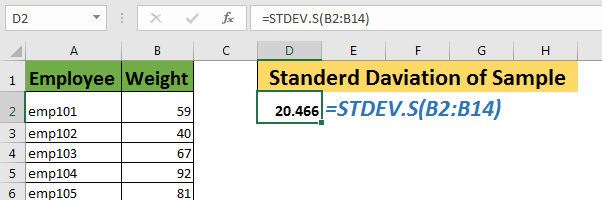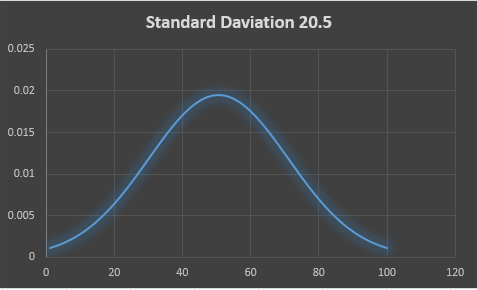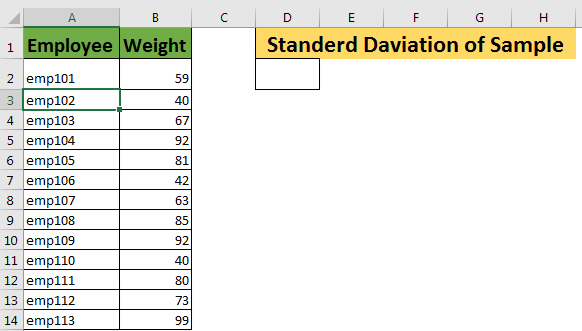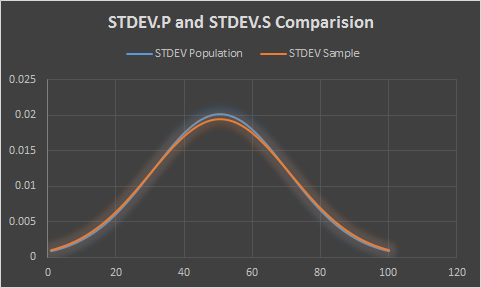# How to use the STDEV.S Function in Excel

The Excel STDEV.S Function is used to calculate the standard deviation in the sample data set. The S in STDEV.S stands for Sample. It is an updated version of STDEVS function in excel. Excel recommends the use of STDEV.S function over STDEVS function. This is also called unbiased analysis.If you want to calculate the standard deviation of the entire population, use STDEV.P function.
What is the standard deviation? It is used to evaluate how far the data is spread from the mean of data sample. It is the squire root of variance.
Syntax of STDEV.S

=STDEV.S(number1,number2,...)

Number1,number2,...: these are the number of which you want to calculate standard deviation.
The first number is compulsory.

The first argument in is compulsory, rest are optional.
These numbers can be supplied as individual numbers, cell references, ranges or arrays to STDEV.S function.
All below formulas are valid STDEV.S formulas.

=STDEV.S(1,2,3,{5,99})
=STDEV.S(A1,A2,B3,C2:C5)

STDEV.S Example
Here we have a data sample of weights of employees. We want to calculate the standard deviation to see how much the weights are spread over the average weights of these employees.Write below STDEV.S formula in cell D2:

=STDEV.S(B2:B14)

This will return standard deviation of around 20.5.If we plot a graph with on 1 to 100 with this standard deviation, the standard deviation graph will look like something this.If we calculate standard deviation using STDEV.P it will be slightly less. It will be 19.7.

The result of STDEV.S always greater than STDEV.P’s result on same data. You can see in below graph that standard deviation of sample is more spread than standard deviation of population.STDEV.S vs STDEV.P
So, what is the difference in STDEV.S and STDEV.P function? Let’s Explore.

• The STDEV.S function is used when your data is a sample of entire population.
• The STDEV.P function is used when your data represents the entire population.
• In STDEV.S or STDEV, the squared deviation is divided by total number of sample -1. It is represented sa N-1.
In STDEV.P function, the squared deviation is divided by total number of arguments, mostly represented as N. that is why, STDEV.P is smaller than STDEV.S. don’t use STDEV.P if you haven’t captured the entire population for analysis.
• The STDEV.S or STDEV function is used on small sample of entire population, we subtract 1 from denominator (number of samples arguments). This is called non-biassed analysis of standard deviation. This is used when analysis is destructive.

Since, STDEV.P function takes whole data, and some factors may dominate the result standard deviation. And Since it will be taken as the standard deviation for everyone in data, even for minorities, this is called Biased Analysis. This is why, this standard deviation is recommended to use only when analysis is non-destructive.
Important:

1. The STDEV.P is just a newer version of STDEVP and STDEV functions of excel. There is no significant difference among them. However Excel recommends use of STDEV.P function
2. The STDEV.P function only recognises numbers. Any other value is ignored.
3. Use this function when you have captured the entire population. For sample data, use STDEV.S function.

So yeah, this STDEV.S function. I hope this was informative. If you have any doubts or suggestion regarding this article or any other function of excel, be my guest and use comment section below. I will be more than happy to here from you guys.

Related Data:
How To Use STDEV.P Function in Excel
How to Use STDEV Function in Excel
Regressions Analysis in Excel
How To Calculate MODE function in Excel
How To Calculate Mean function in Excel
How to Create Standard Deviation Graph

Popular Articles:

50 Excel Shortcuts to Increase Your Productivity

How to use the VLOOKUP Function in Excel

How to use the COUNTIF in Excel 2016

How to use the SUMIF Function in Excel

Terms and Conditions of use

The applications/code on this site are distributed as is and without warranties or liability. In no event shall the owner of the copyrights, or the authors of the applications/code be liable for any loss of profit, any problems or any damage resulting from the use or evaluation of the applications/code.